6.2 Standard Model of Kirton and Uren

Since the McWhorter model suffers from a weak temperature dependence of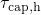and small time constants, Kirton and Uren  incorporated field-independent barriers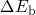in the cross sections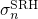and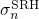(see Section 2.5). The ‘ad hoc’ introduction of these barriers has been motivated by the theory of nonradiative multi-phonon transitions (NMP) process . However, Kirton and Uren have not provided a detailed theoretical derivation based on this NMP theory. Nevertheless, their work is regarded as a substantial improvement in the interpretation of charge trapping at semiconductor-oxide interfaces and thus also referred to as the standard model throughout this thesis. In an extended version of the McWhorter model, the holes can also be captured by traps with an energy below the substrate valence band. As illustrated in Fig. 2.5 of Section 2.5.2, the required barriers consist of two components, namelyand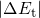. The latter is the required minimum energy for a hole capture process whileis the barrier component which must be overcome for hole capture as well as emission. In this variant, the capture and emission time constants read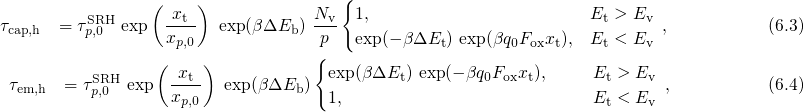where the traps are not restricted to lie within the bandgap. Its behavior with respect to the temperature and the oxide field is illustrated in Fig. 6.1 and evaluated based on the TDDS checklist in Table 6.1. When the trap level lies below the valence band edge (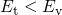),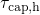shows an exponential field dependence, which is superimposed by a sharp peak due to a drop of the hole concentration at weak oxide fields. Comparing the model to the experimental TDDS data (see Section 1.3.4), this exponential behavior allows for reasonable and approximative fits to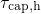but it is incompatible with the observed curvature in. Furthermore, the model predicts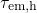to be field insensitive for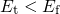according to the equations (6.4). It should be mentioned at this point that the derivation of the analytical expression (6.3) and (6.4) is based on Boltzmann statistics, leading to small deviations inand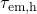compared to the simulations of Fig. 6.1 (left) using Fermi-Dirac statistics. The weak field dependence of the simulatedreasonably agrees with the behavior of ‘normal’ (constant emission times) but is inconsistent with as well as ‘anomalous’ traps (a drop at weak oxide fields). Nevertheless, Fig. 6.1 reveals that the introduction ofyields the required temperature activation and larger time constants in agreement with the points (ii) and (v) of the TDDS findings.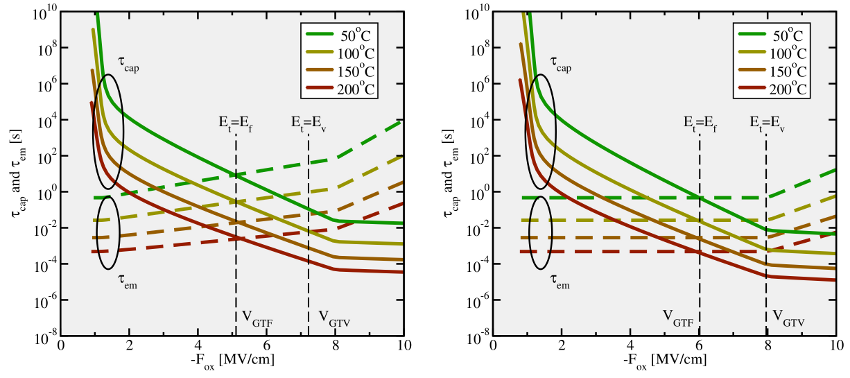Figure 6.1: The simulated time capture and emission times according to the standard model of Kirton et al.   using Fermi-Dirac (left) and Boltzmann (right) statistics. The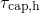are plotted as solid lines, while theare depicted by dashed lines. The vertical dashed lines mark the values of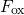or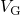when the trap level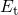passes either the Fermi level or the valence band edge in the substrate, respectively. Equation (6.4), which is based on Boltzmann statistics, predicts that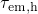remains constant in the region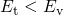as shown in the right figure. Using more accurate Fermi-Dirac statistics the emission time constants are subject to a weak field dependence (cf. left figure). On the logarithmic scale, thefollow a linear behavior in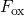over a wide range but do not have the same curvature as present in the TDDS data. Theshow neither a plateau nor a drop towards weak fields. Compared to the McWhorter model,andexhibit a clear temperature activation over the whole range of oxide fields so that their values are moved to larger time scales relevant for NBTI.

A fit of the Kirton model to the experimental TDDS data is presented in Fig. 6.2. Although the model can reproduce some features seen in the TDDS data, except for the curvature in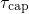, no reasonable agreement with the measurement data could be achieved. This discrepancy can be explained as follows: The exponential bias dependence extends up to a voltageat whichcoincides with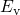. In Fig. 6.2 (left)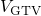is approximately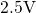so thatshows an exponential bias dependence up to this value and becomes constant afterwards. Therefore,must be chosen such thatlies above the voltage range used in the measurements. This is only the case for defects whose trap levelsare situated sufficiently low. Note that those defects are also characterized by a large, which marks the voltage wherecoincides with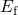. After equation (2.66), theirmust equal theirat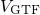, visible as the crossings betweenandin Fig. 6.2. At a low gate bias, their trap levels are moved far below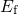so that their emission times fall several orders of magnitude below their corresponding capture time constants. The large difference betweenandpredicted by the Kirton model is inconsistent with the experimental TDDS data. Additionally, a fit of the Kirton model tois presented in Fig. 6.1 (right). It clearly shows that the simulatedfails to reproduce the experimentally obtained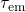when a good match withis achieved.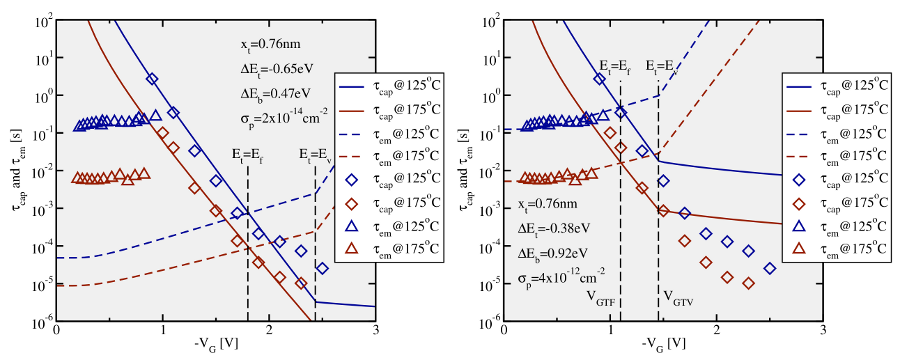Figure 6.2: A fit of the Kirton model to the TDDS data. The symbols stand for the measurement data and the lines represent the simulated time constants. When the Kirton model is optimized to the hole capture times(left), reasonable fits can be achieved for them but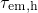is predicted three orders of magnitudes too low. Alternatively, a good agreement (right) can be obtained for the hole emission timesbut with a strong mismatch of the capture timesfor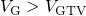. From this it is concluded that the Kirton model is not capable of fittingandat the same time.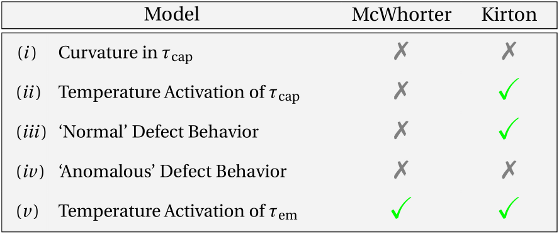Table 6.1: Checklist for a TDDS defect. The individual criteria stem from the TDDS data addressed in Section 1.3.4. The McWhorter as well as the Kirton model do not fulfill all criteria and thus do not describe the defects seen in TDDS experiments.